Courses

# Uni Junction Transistor (UJT) Electrical Engineering (EE) Notes | EduRev

## Electrical Engineering (EE) : Uni Junction Transistor (UJT) Electrical Engineering (EE) Notes | EduRev

The document Uni Junction Transistor (UJT) Electrical Engineering (EE) Notes | EduRev is a part of the Electrical Engineering (EE) Course Electronic Devices.
All you need of Electrical Engineering (EE) at this link: Electrical Engineering (EE)

UNI JUNCTION TRANSISTOR (UJT)

Another device whose construction is similar to that of the FET is shown in the figure.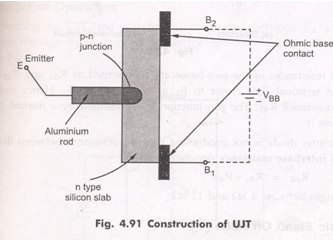It is a three terminal device, having two layers.It consists of a slab of lightly doped N-type silicon material. The two base contacts are attached to both ends of this N-type surface. These are denoted as B1 and B2 respectively.

A P-type material is used to form a pn junction at the boundary of the aluminium rod and N-type slab. The N-type is lightly doped while P-type is heavily doped. That is N-type provides high resistivity and P-type provides low resistivity.

This device was originally described in the literature as the double-base diode, but is now commercially available under the designation Uni Junction transistor (UJT).

The standard symbol for this device is shown in the fig.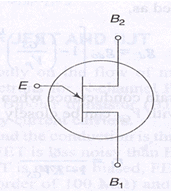Here the emitter arrow is inclined and points toward B1 and This emitter arrow which is at an angle to the vertical line representing N-type material indicates the direction of flow of conventional current when UJT is forward biased.

EQUIVALENT CIRCUIT OF UJT:

The circuit of UJT is shown in the fig.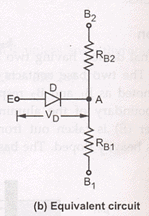In the construction ,the emitter terminal is closer to B2 as compared to B1.

If we see the equivalent circuit of UJT, the internal resistances of the two bases are represented as Rb1 and Rb2. Hence Rb1 is greater than Rb2. The pn junction is represented by a normal diode with Vd  as the drop across it. When the emitter diode is not conducting then the resistance between the two bases Band B2 is called interbase resistance denoted by Rbb.

Rbb=Rb1+Rb2

Its value ranges from 4kΩ to 12kΩ.

Intrinsic stand off ratio (η)

Consider UJT as shown in the fig. to which supply Vbb is connected. With Ie=0, That is emitter diode is not conducting ,

Rbb=Rb1+Rb2

Then the voltage drop across Rb1  can be obtained by using potential divider rule: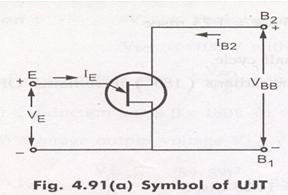Replacing with its equivalent circuitRbb=Rb1+Rb2

When Ie =0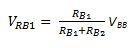= η.VBB  When Ie=0

η = Intrinsic stand off ratio =  (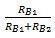) when Ie=0

η = (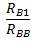) when Ie=0

The value of η is from 0.5 to 0.8 the voltage Vrb1 is called intrinsic stand off voltage, because it keeps the emitter diode reverse biased for all emitter voltages less than Vrb1.

Operation: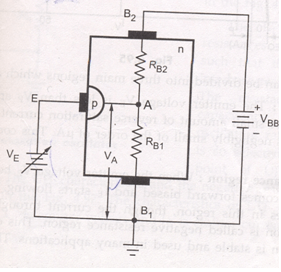1.The supply Vbb is applied between B2 and B.

2. Variable emitter voltage Ve  is applied across the emitter terminals.

3. If V is varied , potential of A is decided by η and is equal to ηVbb

Va=Vrb1= ηVbb

Case 1:  If Ve< Va

As long as V< Va, the pn junction is reverse biased. Hence emitter current Iwill not flow. Thus, UJT is said to be off.

Case 2: if Ve>Vp

The diode drop V is generally between 0.3 to 0.7v.

We can write Vp=Va+Vd = ηVbb+Vd

When Vbecomes equal to or greater than Vp, the pn junction becomes forward biased and current Ie flows. Thus, UJT  is said to be  ON.

VI Characteristics:

1.The graph of IE against emitter voltage plotted for a particular value of Vbb is called the characteristics of UJT.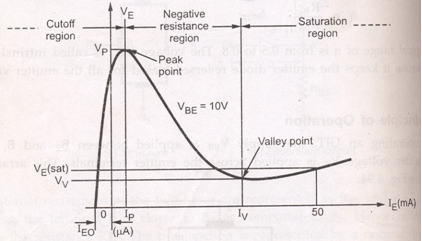The characteristics can be divided in to three main regions.

1.cut off     2.  Negative resistance region   3.  Saturation region.

1.cut off:   When Ve< Vp and the pn junction is reverse biased. A small amount of reverse saturation current IEO flows through the device which is negligibly small of the order of micro amps.  This condition remains till the peak point.

2. Negative resistance region: When emitter voltage VE  becomes equal to Vp then pn junction becomes forward biased and IE starts flowing. The voltage across the device decreases in this region though the current through the device increases. Hence the region is called negative resistance region. This decreases the resistance Rb1. This region is stable and used in many applications. This region continues till valley point.

3. Saturation region:   The region to the right of the valley point is called saturation region. In the valley point, the resistance changes from negative to positive. The resistance remains positive in the saturation region.

As Vbb increases, the potential Vp corresponding to peak point will increase.

Applications of UJT:

The  UJT is mainly used in the triggering of other devices such as SCR. It is also used in the sawtooth wave generators and some timing circuits. The most popular application of UJT is as a relaxation oscillator to obtain short pulses for triggering of SCRs.

The relaxation oscillator using UJT which is meant for generating sawtooth waveform consists of a UJT and a capacitor, which is charged through emitter resistor as the supply voltage Vbb is switched ON.

Offer running on EduRev: Apply code STAYHOME200 to get INR 200 off on our premium plan EduRev Infinity!

,

,

,

,

,

,

,

,

,

,

,

,

,

,

,

,

,

,

,

,

,

;